# Enthalpy

In thermodynamics, enthalpy (also known as thermodynamic potential) is the sum of the internal energy U and the product of pressure p and volume V of a system,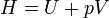$H = U + pV \,$

This characteristic function used to be called "heat contents", which is why it is conventionally indicated by H. The term "enthalpy" was coined by the Dutch physicist Heike Kamerling Onnes.

The internal energy U and the work term pV have dimension of energy, in SI units this is joule; the extensive (linear in size) quantity H has the same dimension.

Enthalpy (as the extensive property mentioned above) has corresponding intensive (size-independent) properties for pure materials. A corresponding intensive property is specific enthalpy, which is enthalpy per mass of substance involved. Specific enthalpy is denoted by a lower case h, with dimension of energy per mass (SI unit: joule/kg). If a molecular mass or number of moles involved can be assigned, then another corresponding intensive property is molar enthalpy, which is enthalpy per mole of the compound involved, or alternatively specific enthalpy times molecular mass. There is no universally agreed upon symbol for molar properties, and molar enthalpy has been at times confusingly symbolized by H, as in extensive enthalpy. The dimensions of molar enthalpy are energy per number of moles (SI unit: joule/mole).

In terms of intensive properties, specific enthalpy can be correspondingly defined as follows: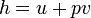$h = u + p v\,$

where

h = specific enthalpy
u = specific internal energy
p = pressure (as before)
v = specific volume = reciprocal of density

Enthalpy is a function depending on the independent variables that describe the state of the thermodynamic system. Most commonly one considers systems that have three forms of energy contact with their surroundings, namely the reversible and infinitesimal gain of heat, DQ = TdS, loss of energy by mechanical work done by the system −pdV, and acquiring of substance, μ dn. The states of systems with three energy contacts are determined by three independent variables. Although a fairly arbitrary choice of three variables is possible, it is most convenient to consider H(S,p,n), that is, to describe H as function of its "natural variables" entropy S, pressure p, and amount of substance n.

In thermodynamics one usually works with differentials. In this case$dH = dU + pdV + Vdp \,$

The internal energy dU and the corresponding enthalpy dH are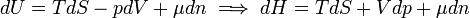$dU = TdS - pdV + \mu dn \;\Longrightarrow\; dH = TdS + Vdp +\mu dn$

The rightmost side is an equation for the characteristic function H in terms of the natural variables S, p, and n.

The first law of thermodynamics can be written—for a system with constant amount of substance—as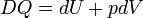$DQ = dU + pdV \,$

If we keep p constant (an isobaric process) and integrate from state 1 to state 2, we find$\int_1^2 DQ = \int_1^2 dU + \int_1^2 pdV \;\Longrightarrow\; Q = U_2 - U_1 + p(V_2-V_1) = (U_2 +pV_2) - (U_1 + pV_1) = H_2 - H_1 = \int_1^2 dH,$

where symbolically the total amount of heat absorbed by the system, Q, is written as an integral. The other integrals have the usual definition of integrals of functions. The final equation (valid for an isobaric process) is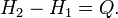$H_2-H_1 = Q. \,$

In other words, if the only work done is a change of volume at constant pressure, W = p(V2V1), the enthalpy change H2H1 is exactly equal to the heat Q transferred to the system.

As with other thermodynamic energy functions, it is neither convenient nor necessary to determine absolute values of enthalpy. For each substance, the zero-enthalpy state can be some convenient reference state.